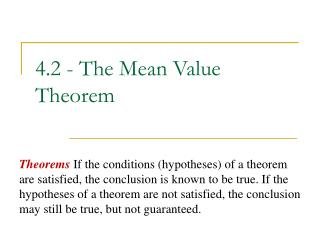# 4.2 - The Mean Value Theorem - PowerPoint PPT PresentationDownload Presentation4.2 - The Mean Value Theorem

4.2 - The Mean Value Theorem
Download Presentation## 4.2 - The Mean Value Theorem

- - - - - - - - - - - - - - - - - - - - - - - - - - - E N D - - - - - - - - - - - - - - - - - - - - - - - - - - -
##### Presentation Transcript

1. 4.2 - The Mean Value Theorem TheoremsIf the conditions (hypotheses) of a theorem are satisfied, the conclusion is known to be true. If the hypotheses of a theorem are not satisfied, the conclusion may still be true, but not guaranteed.

2. Rolle’s Theorem • Let f be a function that satisfies the following three hypotheses: • f is continuous on the closed interval [a, b]. • f is differentiable on the open interval (a, b). • f (a) = f (b) • Then there is a number c in (a, b) such that • f′(c) = 0.

3. Examples: Rolle’s Theorem • Explain why the conclusion to Rolle’s Theorem is not guaranteed for the function • f (x) = x / (x – 3) on the interval [1, 6]. 2. Verify that the function satisfies the three hypotheses of Rolle’s Theorem on the given interval. Then find all numbers c that satisfy the conclusion of Rolle’s Theorem.

4. The Mean Value Theorem • Let f be a function that satisfies the following two hypotheses: • f is continuous on the closed interval [a, b]. • f is differentiable on the open interval (a, b). • Then there is a number c in (a, b) such that

5. Example: Mean Value Theorem 3. Verify that the function satisfies the two hypotheses of Mean Value Theorem on the given interval. Then find all numbers c that satisfy the conclusion of Mean Value Theorem.

6. Theorem If f ′(x) = 0 for all x in an interval (a, b), then f is constant on (a, b). Corollary If f ′(x) = g′(x) for all x in an interval (a, b), then f – g is constant on (a, b); that is, f(x) = g(x) + c where c is a constant. Example: If f (x) = x2 + 3 and g(x) = x2 + 7, find f ′(x), g ′(x), and (f – g)(x).

7. Proof By Contradiction • Assume that something is true. • Show that under your assumptions, the conditions of a known theorem are satisfied. This guarantees the conclusion of that theorem. • Show that the conclusion of the theorem is, in fact, not true under the assumptions. • Since the conclusion of the theorem must be true if the assumptions were satisfied, the only conclusion left is that the assumptions must be incorrect.

8. Example Show that the equation 2x – 1 – sin x = 0 has exactly one real root.